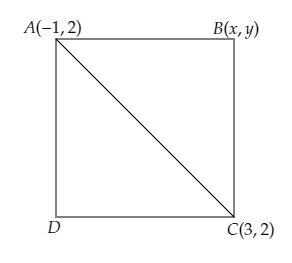# Two opposite vertices of a square are $(-1, 2)$ and $(3, 2)$. Find the coordinates of other two vertices.

Given:

Two opposite vertices of a square are $(-1, 2)$ and $(3, 2)$.

To do:

We have to find the coordinates of other two vertices.

Solution:

Let ABCD be the given square and $A (-1, 2)$ and $C (3, 2)$ are the opposite vertices.
Let the coordinates of $B$ be $(x, y)$. Join AC.This implies,

$AB=BC=CD=DA$

We know that,

The distance between two points $\mathrm{A}\left(x_{1}, y_{1}\right)$ and $\mathrm{B}\left(x_{2}, y_{2}\right)$ is $\sqrt{\left(x_{2}-x_{1}\right)^{2}+\left(y_{2}-y_{1}\right)^{2}}$.

Therefore,

$\mathrm{AB}=\mathrm{BC}$

Squaring on both sides, we get,

$\mathrm{AB}^{2}=\mathrm{BC}^{2}$

$\Rightarrow (x+1)^{2}+(y-2)^{2}=(x-3)^{2}+(y-2)^{2}$

$\Rightarrow x^{2}+2 x+1+y^{2}-4 y+4 =x^{2}-6 x+9+y^{2}-4 y+4$

$\Rightarrow 2 x+6 x-4 y+4 y=13-5$

$\Rightarrow 8 x=8$

$\Rightarrow x=1$.........(i)

$\mathrm{ABC}$ is a right angled triangle.

$\Rightarrow \mathrm{AC}^{2}=\mathrm{AB}^{2}+\mathrm{BC}^{2}$

$\Rightarrow (3+1)^{2}+(2-2)^{2}=x^{2}+2 x+1+y^{2}-4 y +4+x^{2}-6 x+9+y^{2}-4 y+4$

$(4)^{2}=2 x^{2}+2 y^{2}-4 x-8 y+18$

$16=2 x^{2}+2 y^{2}-4 x-8 y+18$

$\Rightarrow 2 x^{2}+2 y^{2}-4 x-8 y+18-16=0$

$\Rightarrow 2 x^{2}+2 y^{2}-4 x-8 y+2=0$

$\Rightarrow 2 (x^{2}+y^{2}-2 x-4 y+1)=0$

$\Rightarrow x^{2}+y^{2}-2x-4y+1=0$

Substituting $x=1$, we get,

$(1)^{2}+y^{2}-2(1)-4 y+1=0$

$\Rightarrow 1+y^2-2-4y+1=0$

$\Rightarrow y^2-4y=0$

$\Rightarrow y(y-4)=0$

$\Rightarrow(y)(y-4)=0$

$y=0$ or $y-4=0$

$y=0$ or $y=4$

Therefore, the other points of the square are $(1,0)$ and $(1,4)$.